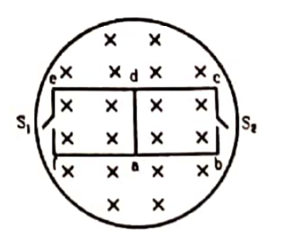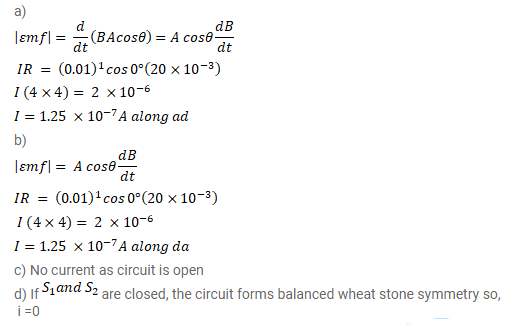# The magnetic field in the cylindrical shown in figure increases at

Question:

The magnetic field in the cylindrical shown in figure increases at a constant rate of $20.0 \mathrm{mT} / \mathrm{s}$. Each side of the square loop abcd and defa has a length of $1.00 \mathrm{~cm}$ and a resistance of $4.00 \Omega$. Find the current (magnitude and sense) in the wire ad if (a) the switch $S_{1}$ is closed but $S_{2}$ is open, (b) $S_{1}$ is open but $S_{2}$ is closed, (c) both $S_{1}$ and $S_{2}$ are open and (d) both $S_{1}$ and $S_{2}$ are closed.Solution: